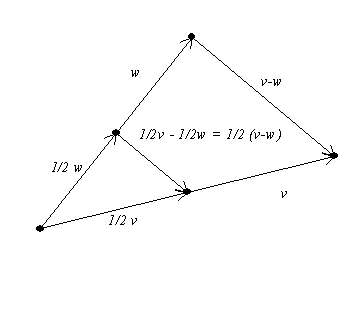Proposition: The segment joining the midpoints of two sides of a triangle is parallel to the third side and is 1/2 of the measure of the third side.

A vector proof:
Suppose the two side correspond to the position vectors, v and w. so the midpoints correspond to the vectors 1/2 v and 1/2 w.The line segment joining the midpoints of two sides corresponds to the vector, z = 1/2w -1/2v = 1/2 (w-v).
Since z is a scalar multiple of w-v, it is parallel  to w-v, the vector determined by the third side of the triangle.
The scalar is 1/2 , so z has a length 1/2 of the vector w-v. IRMC.